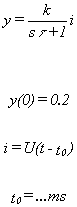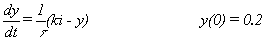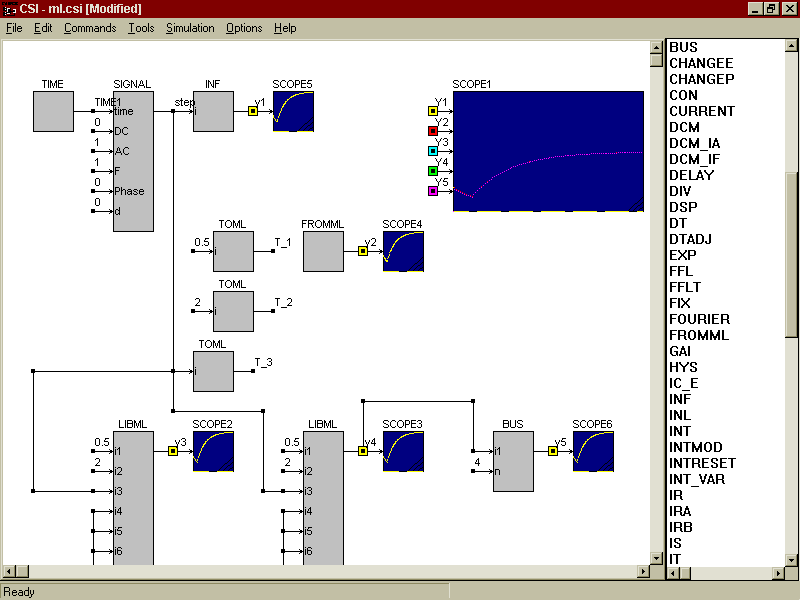• Introduction
• What is in this manual
• What is Caspoc
• User interface
• Introduction
• Starting
• Simulation
• Editing
• Viewing and printing
• Getting Started
• Basic editing
• Simulation in the time domain
• Basic User Interface Topics
• Editing
• Simulation
• Viewing
• Library
• Reports
• Project management
• Circuit and Block Diagram Components
• Introduction
• Cscript and user defined functions
• Component parameters
• Modeling Topics
• Introduction
• Power Electronics
• Semiconductors
• Electrical Machines
• Electrical drives
• Power Systems
• Mechanical Systems
• Thermal Systems
• Magnetic Circuits
• Green Energy
• Coupling to FEM
• Experimenter
• Analog hardware description language
• Embedded C code Export
• Coupling to Spice
• Small Signal Analysis
• Matlab coupling
• Tips and tricks
• Appendices

## MODELING LANGUAGE PASCAL

The main advantage of the modeling language is that the model (in modeling language) is compiled into an executable Dynamic Link Library (DLL), which is executed directly by the computer.
The compilation of the modeling language model into the executable code can be performed by any commercial available compiler, which can generate a dynamic link library (DLL) executable file. Since the dll executable file consists of moveable code, it is only available for Windows 95/98/NT.
In this paragraph Delphi 3.0TM is chosen to construct the modeling language dll executable. As example a model of a first order differential equation is selected and described.where i is a step function. The differential equation is rewritten intoThe first order differential equation is modeled in the modeling language using Pascal, as shown in figure for the modeling language. The block diagram model is shown which is the interface with the modeling language. To show the possibilities of the modeling language the first order differential equation is accessed 4 times in the block diagram model.

1. In the block diagram model by using the block 'INF'. The output from the block 'INF' is y1
2. As Standard Modeling Language by the file ml.dll, which is loaded in the menu 'Files' and interfacing using the blocks ToML and FromML. The output from the Standard Modeling Language is y2
3. ,
4. As Library Modeling Language block, the model can be used multiple times in the block diagram. To demonstrate this the output y3 and y4 are both modeled by a LibML block.The resulting 4 outputs are graphically displayed.
The Pascal file for the Standard Modeling Language is equal to the Pascal file for the Library Modeling Language. First the syntax of this Pascal file is discussed, second the differences between the Standard Modeling Language and the Library Modeling Language are discussed.
The Pascal file for the modeling language is displayed and consists of a number of distinct parts such as the

 Section Lines Library Name 1 Local variable declarations 15 to 17 Export section 42 to 43

You always have to use this structure to set up a modeling language file.
Library Name
The name of the DLL file is inserted after the keyword LIBRARY in line 1. Use the same name as the MSDOS file name *.pas and *.dll.
Line 2 to 14 show the header of the modeling language. The header indicates the start of a function in the modeling language. The name of the function is inserted after the keyword Function in line 2. This function name has to be included in the export section of the modeling language, see line 42 and 43. For standard modeling language the function name should be StandardML. For Library Modeling Language any name can be chosen. In this example the name StandardML is chosen to make the file compatible between Standard Modeling Language and Library Modeling Language. In this way the ML.DLL file can be accessed as Standard Modeling Language using ToML blocks, or as Library Modeling Language using the LibML block.
In the header the variables for data interfacing to the block diagram are declared. The common type is an array of extended. Only the address of this array is passed through the header, indicated by the keyword var in the lines 3 to 13.
Inputs of the block LibML for Library Modeling Language. For Standard Modeling Language this array is a copy of the array bus
Inputs for the standard modeling language via the block ToML and output for the Standard Modeling Language via the block FromML. The array bus serves as a storage array for both the Standard Modeling Language and the Library Modeling Language.
You can build a counter based on the array bus like
bus:=bus+1;
Here bus variable number 3; bus is used as counter. Also a shift register can be based on the array bus. You can store sampled data in the array using the following code

       If Trigger>0 then           Begin           For i:=n Downto 2 Do                bus[i]:=bus[i-1];           bus[i]:=New_Sample;           End; 

Initial conditions for the differential equations in the modeling language.
State variable array for the differential equations in the modeling language.

Derivative of the state variable array for the differential equations in the modeling language.
Reserved arrays for future version of modeling language in CASPOC.
Simulation parameters from CASPOC are available in the modeling language via the array p. In version 1.6 the following parameters are defined
p Procedure number for the Standard Modeling Language as defined in the ToML and FromML blocks. p=0 indicates the initialization of the modeling language.
p Simulation time t.
p Integration time step dt.
Reserved arrays for future version of modeling language in CASPOC.
Line 14 indicates the end of the header. The output of the function is of the type extended and is set as the output of the block LibML. The keyword EXPORT; indicates that the function is exported, see also line 43.
Local variable declarations
In line 15, 16 and 17 local variables are defined. all variables for algebraic operations involving the arrays from the header have to be declared of the type extended. Notice that the local variables loose their content after leaving the function in the modeling language. Therefore you can use them only for intermediate calculations within a function.
Main function
The code for the modeling language starts with Begin in line 18 and ends with End; in line 41.
The Case statement is used to identify different codes for initialization indicated by p=0 and different procedure numbers indicated by p. In this example only procedure number 1 is used as indicated in line 27. In the ToML and FromML blocks in the block diagram only procedure number 1 is used for the Standard Modeling Language. In the case of Library Modeling Language p=0 for the initialization. During the rest of the simulation p=1 for the Library Modeling Language.
Initialization of the bus variables is done directly on the array bus. Initialization of the differential equations is performed on the array x0.
The inputs for the LibML blocks are stored in the array Input for the Library Modeling Language. For the Standard Modeling Language the inputs defined in the block ToML are stored in the array bus. Before a call to the Standard Modeling Language the array bus is copied to the array input. In this way there is compatibility between the Standard Modeling Language and the Library Modeling Language. You can't use the array input for storage of data.
The actual model, the first order differential equation is modeled at line 32. Notice that the array dxdt can only be placed at the left side of the := symbol.
The string at line 36, stored in m, will appear in the menu 'Data', item 'Bus variables'.
The output for the Library Modeling Language of the block LibML is defined in line 40.
Export
The keyword Exports is used to export the name of the function in the dynamic link library file. In this way CASPOC can identify your function in the modeling language. Include here the names of the functions you defined in the headers.
End
Include a Begin End. Notice in the Pascal file to indicate the end of the library file, see line 44 and 45.
You can include more functions in a library. Each function should have its own header and main function as indicated by the lines 2 to 41.
The keyword Exports is defined only once. Each name of a function has to be included after the keyword exports.
Only the function with the name StandardML can be used for Standard Modeling Language.

 * Definition of the step function t          TIME K          CON      0.5 tau        CON      2 step       SIGNAL   t   0 1 1 0 0 0 1 5 * Block diagram model *                          y(0)  K     tau y1         INF      step   0.2   0.5   2 * Standard Modeling Language T_1        ToML     K      1  1 T_2        ToML     tau    1  2 T_3        ToML     step   1  3 y2         FromML          1  4 * Library Modeling Language y3         LibML    data/ml/ml.dll   StandardML(K,tau,step) y4         LibML    data/ml/ml.dll   StandardML(K,tau,step) * Value of bus after a call to LibML by y3 bus4       BUS      y3  4    .end 

  { 1}     LIBRARY  ML; ; uses  SysUtils,  Classes; { 2}     FUNCTION StandardML( { 3}                         VAR input           : ARRAY OF EXTENDED; { 4}                         VAR bus             : ARRAY OF EXTENDED; { 5}                         VAR x0              : ARRAY OF EXTENDED; { 6}                         VAR x               : ARRAY OF EXTENDED; { 7}                         VAR dxdt            : ARRAY OF EXTENDED; { 8}                         VAR reserve1        : ARRAY OF EXTENDED; { 9}                         VAR reserve2        : ARRAY OF EXTENDED; {10}                         VAR reserve3        : ARRAY OF EXTENDED; {11}                         VAR reserve4        : ARRAY OF EXTENDED; {12}                         VAR p               : ARRAY OF EXTENDED; {13}                         VAR m               : ARRAY OF STRING {14}                        ):EXTENDED;EXPORT; {15}     VAR K,tau,step      : EXTENDED; {16}     VAR ProcedureNumber : INTEGER; {17}     VAR t,dt            : EXTENDED; {18}     begin {19}     ProcedureNumber := TRUNC(p); {20}     t               := p; {21}     dt              := p; {22}     CASE ProcedureNumber OF {23}       0:BEGIN {24}         x0  := 0.2; {25}         bus := 0.2; {26}         END; {27}       1:BEGIN {29}         K      := input; {30}         tau    := input; {31}         step   := input; {32}         dxdt:= (1/tau) * (k*step-x); {33}         bus := x; {34}         END; {35}       END; {36} {37} {38} {39} {40}     StandardML := bus; {41}     END; {42}     EXPORTS {43}     StandardML; {44}     BEGIN {45}     END.